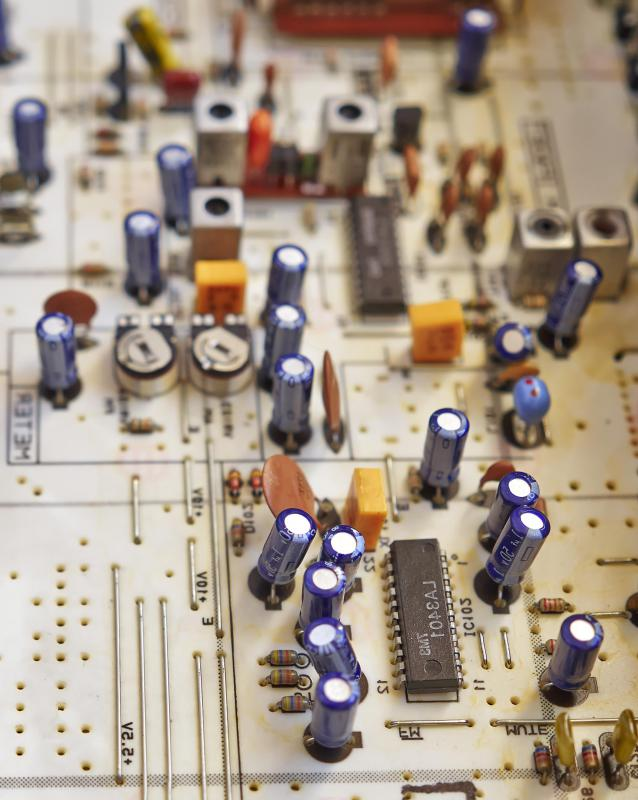# What is a Linear Integrated Circuit? (with picture)

Jean Marie Asta
Jean Marie AstaCommonly found in various type of circuits, resistors are a type of electronic component that can lower a circuit’s voltage and its flow of electrical current.

A linear integrated circuit is used in a variety of modern electronic equipment. The circuit is able to receive, process and produce a variety of different levels of energy as the device operates. Devices that require amplifiers and oscillators often make use of this type of circuit, which is characterized by equal input and output signal levels.

To understand what a linear integrated circuit is, it's important to first understand what an integrated circuit, or IC, is. An IC is probably better known as a chip or microchip. It is a type of semiconductor that has a series of resistors, capacitors, and transistors built into it. Each IC can have hundreds or millions of these. Integrated circuits are often used as microprocessors, computer memory, amplifiers, oscillators or timers.

These circuits can be either analog or digital. Linear integrated circuits are analog ICs. They are different than digital ICs because they are capable of creating a large variety of levels of output. In fact, in theory, this circuit should be capable of providing an infinite number of different signal levels. A digital integrated circuit, in contrast, is able to produce only a few different levels of output.

Analog ICs are called linear integrated circuits because the signal output level of the circuit is a linear function of the signal input level. Graphing the input and output levels demonstrates this fact visually. If the output is graphed at the same moment as the input, connecting the points would produce a straight line. In other words, as the input changes, the output of the circuit changes proportionately.

Linear integrated circuits are used for functions where the signal output needs to vary such as for audio-frequency and radio-frequency amplifiers. Devices like audio amplifiers, DC amplifiers, oscillators and multivibrators use these circuits. The most common type of linear integrated circuit is an operational amplifier, or op amp, which consists of a conventional analog circuit formed with transistors, resistors and diodes. In an op amp, there are two different inputs, one of which is inverting and one of which is non-inverting.

When a signal is applied at the inverting input, a corresponding and opposite phase is produced at the output. Applying a signal to the non-inverting input of the circuit results in an identical phase produced at the output. Variable resistance creates a connection between the inverting input and the output, which controls the amplification of the signal.

## You might also Like